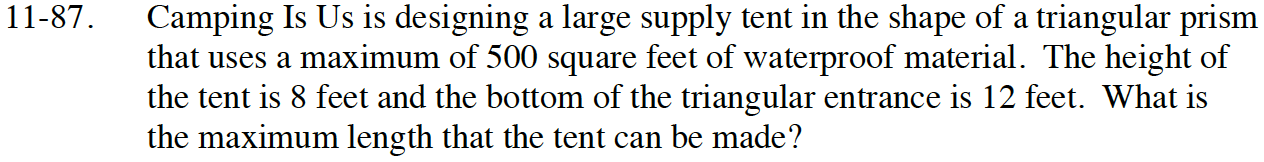Home > INT3 > Chapter 11 > Lesson 11.2.3 > Problem11-87

11-87.

Camping Is Us is designing a large supply tent in the shape of a triangular prism that uses a maximum of 500 square feet of waterproof material. The height of the tent is 8 feet and the bottom of the triangular entrance is 12 feet. What is the maximum length that the tent can be made? Homework Help ✎Draw a diagram.

Find the area of the two triangular end pieces.

Find the width of the two side pieces.

If x is the length of the tent, write an equation showing that the sum of the five sides must be ≤ 500.

x = 12.625
So probably 12.5 or 12 feet.